# Because Coin Collectors like puzzles, can you answer this?

edited January 31, 2021 6:14AM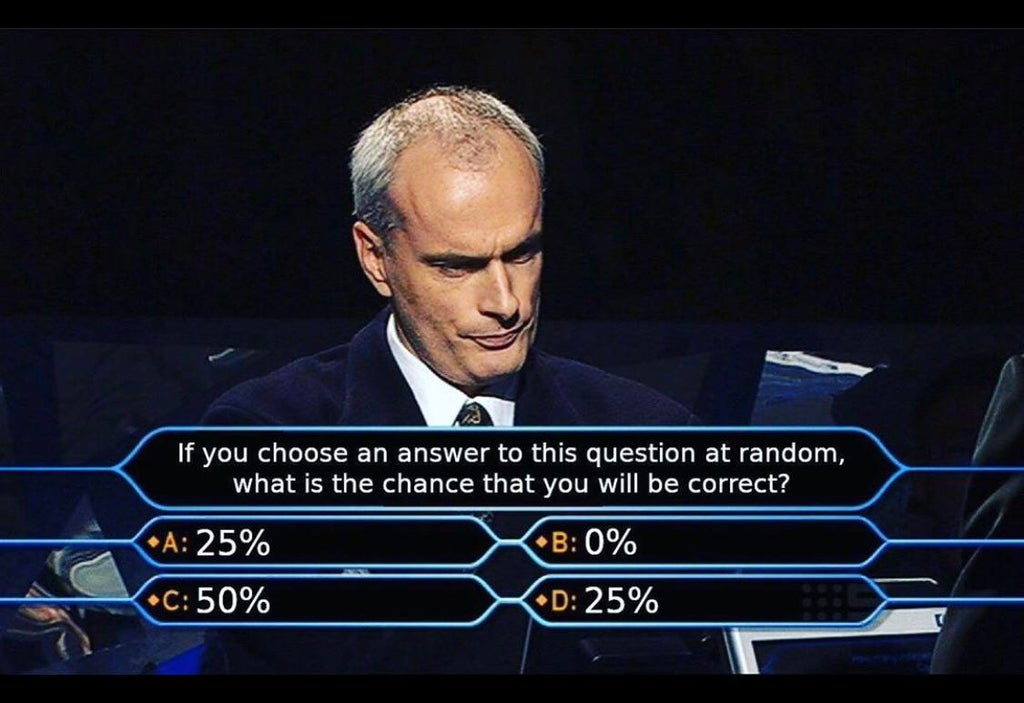Taking a stab at this...
The chance of selecting One answer is 25%.
Chance of selecting the particular answers are:
"25%" is a 2/4 or 50% chance.
"0%" is 1/4 or 25% chance.
"50%" is 1/4 or 25% chance.

-A Final Answer OR D Final Answer: Assume the Correct answer is 25% (i.e., you would be correct if you randomly chose A or D)
-Your random chance of selecting the 25% answer is 2/4 or 50%, so if 25% is the chance of being correct randomly, but you'll get that same answer 50% of the time choosing randomly, that's a contradiction, and they can't simultaneously be true.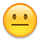• zero percent ??

Successful transactions with : MICHAELDIXON, Manorcourtman, Bochiman, bolivarshagnasty, AUandAG, onlyroosies, chumley, Weiss, jdimmick, BAJJERFAN, gene1978, TJM965, Smittys, GRANDAM, JTHawaii, mainejoe, softparade, derryb

Bad transactions with : nobody to date

• I’ll answer E.Spoiler alert: There’s a good write up of this puzzle here: https://bitmingw.com/2016/06/19/the-multi-choice-paradox/

• @1630Boston said:
zero percent ??

"B. Final Answer" aka assume the correct answer is 0% (you would be correct only if you randomly chose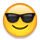.
Your random chance of selecting B is 25%, and if 0% is the right answer, you must have a 0% chance of randomly selecting the right answer. Yet another contradiction, and there is no state where any of 0, 25, or 50% can simultaneously be the correct Random chance of selecting the answer and the correct answer itself.

• 25%

• This site is great. A few weeks back we had the Monte Hall problem come up and now this one. Keep the math coming.

I too will go off the menu and select E All of the above. The best items are often not on the menu.

• If you can answer 25% without having to pick the letter then there only 3 possible answers (25%, 0%, 50%) so it’s 33.3333333%. But if the alphabet letter has to be declared then there are 4 unique answers (A25, B0, C50, D25) because A doesn’t equal D and it’s 25%.

Mr_Spud

• Honey...where's my crazy 8 ball? Peace Roy

BST: endeavor1967, synchr, kliao, Outhaul, Donttellthewife, U1Chicago, ajaan, mCarney1173, SurfinHi, MWallace, Sandman70gt, Ricko, mustanggt, Pittstate03, Lazybones, Walkerguy21D, coinandcurrency242 , thebigeng, Collectorcoins, JimTyler, USMarine6, Elkevvo, Coll3ctor, Yorkshireman, CUKevin, ranshdow, Jzyskowski1, CoinHunter4, bennybravo, Centsearcher, braddick, Windycity, ZoidMeister, mirabela, JJM, RichURich

• 50%. 0% is not possible. The choices left are 25% and 50%. Therefore you have a 50-50 chance of getting the correct answer.

• You have a 50% chance.

• Are you guys saying the random chance of a correct response is 50%, or is your answer selection "C"?

• You should read the link I posted above. The problem isn’t quite as easy as it seems on the surface.

• I think there is no correct answer.

0% is not correct because if there is a 25% chance of picking it. So it is not 0%.

25% is not correct because there are two 25% values and the chance of picking one is 50%. So it is not 25%.

50% is not correct because I just proved that it can't be 0% or either of the two 25% amounts. So it is not 50%.

Therefore, there is no correct answer.

An authorized PCGS dealer, and a contributor to the Red Book.

• @RichieURich said:
I think there is no correct answer.

0% is not correct because if there is a 25% chance of picking it. So it is not 0%.

25% is not correct because there are two 25% values, and the chance of picking one is 50%. So it is not 25%.

50% is not correct because I just proved that it couldn't be 0% or either of the two 25% amounts. So it is not 50%.

Therefore, there is no correct answer.

• Maybe 25% as it is actually 50% and there is a 25% chance of picking 50%.

• @abcde12345 said:

@RichieURich said:
I think there is no correct answer.

0% is not correct because if there is a 25% chance of picking it. So it is not 0%.

25% is not correct because there are two 25% values, and the chance of picking one is 50%. So it is not 25%.

50% is not correct because I just proved that it couldn't be 0% or either of the two 25% amounts. So it is not 50%.

Therefore, there is no correct answer.

But there is a 25% chance of selecting "B" at random so 0% can't be correct.

An authorized PCGS dealer, and a contributor to the Red Book.

• In a coin related format: If you could choose an 1881S Morgan dollar at random, what’s the chance it would be the nicest coin?
A. PCGS MS64 CAC GOLD
B. PCGS MS65 CAC GREEN
C. PCGS MS66 NO CAC
D. NGC MS67 NO CAC

• A and D are the same answer. B and C are two different answers. Choosing an answer is 1 out of 4 or 25%. Getting the answer correct would be 1 out of 3 or 33.33%. I think,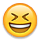"May the silver waves that bear you heavenward be filled with love’s whisperings"

"A dog breaks your heart only one time and that is when they pass on". Unknown
• My brain hurts.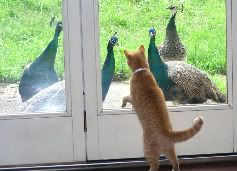• Can't answer the question at this time.
It is Sunday afternoon. Re-watching the playoffs.
Drinking has commenced at 12 noon ( really during the pregame show).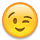Kennedys are my quest...

• 0% is correct answer if you choose any.
So, 25% chance.

• So, WHAT is the question??????????????????????

Today is the first day of the rest of my life
• @1Mike1 said:
A and D are the same answer. B and C are two different answers. Choosing an answer is 1 out of 4 or 25%. Getting the answer correct would be 1 out of 3 or 33.33%. I think,I agree! So are we correct? 🤣😂

• @WQuarterFreddie said:

@1Mike1 said:
A and D are the same answer. B and C are two different answers. Choosing an answer is 1 out of 4 or 25%. Getting the answer correct would be 1 out of 3 or 33.33%. I think,I agree! So are we correct? 🤣😂

Maybe (25%). Maybe not (75%).